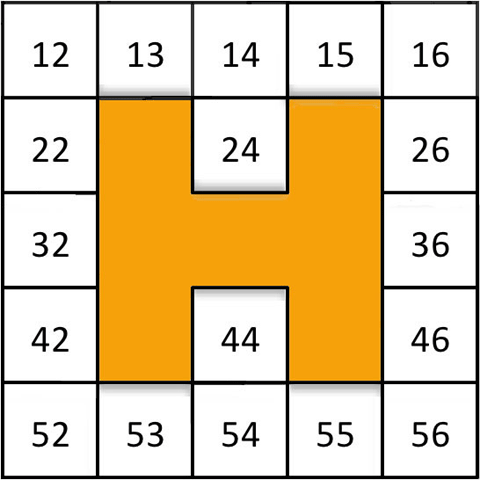# W questions covered by H

In the Big Ideas section of this resource, there is an activity called Covered by H.Numbers covered by H.

Here is part of the dialogue that took place when a year 4 teacher used some W questions with the H-shape provided.

 Teacher: What makes you think the first box is 23? Student: It comes after 22. Teacher: So what if it was in this row? Tell me what you are thinking. Student: All that row starts with 20 and this starts with 30 so it would be 33. Teacher: What stayed the same, and why? Student: The three because that row [pointing vertically] is all threes. Teacher: Three… Student: Units? Units. Teacher: And where would the five units column be? [Student points] Good, so what can you tell me about the horizontal rows — across like this — and the vertical columns like this? How could you describe the rule, the pattern? Student: Horizontal, across, they go up by ones, in order. Tens is vertical, vertical, down, goes up by tens: 15, 25, 35, 45. Teacher: Okay. So what if this vertical column was 115, instead of 15. Where would 135 be, and why? Student: 135. Okay. So that's 115, 125, 135 [pointing correctly]. Teacher: Good. Why?